# Package usage

This vignette summarizes the functionality of the survex package by comparing two survival models. A Cox Proportional Hazards model is analysed alongside a Random Survival Forest, showcasing the functionality of the explanations and finding differences and similarities between the two models.

# Model and explainer creation

For the purpose of this presentation, the veteran dataset from the survival package will be used. The first step is the creation of the models that will be used for making predictions and their explainers.

It is important to note that for the explain() function to be able to extract the data automatically, we either have to set certain parameters while creating the coxph model or provide them manually. We chose to set the required parameters. The creation of an explainer for the random survival forest doesn’t require any additional steps.

library(survex)
library(survival)

set.seed(123)

vet <- survival::veteran

cph <- coxph(Surv(time, status)~., data = vet, model = TRUE, x = TRUE)
cph_exp <- explain(cph)
#> Preparation of a new explainer is initiated
#>   -> model label       :  coxph ( [33m default [39m )
#>   -> data              :  137  rows  6  cols (  extracted from the model  )
#>   -> target variable   :  137  values ( 128 events and 9 censored ) (  extracted from the model  )
#>   -> times             :  94 unique time points , min = 1 , mean = 119.9706 , max = 845.56
#>   -> times             :  (  generated from y with method quantiles  )
#>   -> predict function  :  predict.coxph with type = 'risk' will be used ( [33m default [39m )
#>   -> predict survival function  :  predictSurvProb.coxph will be used ( [33m default [39m )
#>   -> predict cumulative hazard function  :  -log(predict_survival_function) will be used ( [33m default [39m )
#>   -> model_info        :  package survival , ver. 3.3.1 , task survival ( [33m default [39m )
#>   A new explainer has been created!

rsf <- randomForestSRC::rfsrc(Surv(time, status)~., data = vet)
rsf_exp <- explain(rsf)
#> Preparation of a new explainer is initiated
#>   -> model label       :  rfsrc ( [33m default [39m )
#>   -> data              :  137  rows  6  cols (  extracted from the model  )
#>   -> target variable   :  137  values ( 128 events and 9 censored ) (  extracted from the model  )
#>   -> times             :  94 unique time points , min = 1 , mean = 119.9706 , max = 845.56
#>   -> times             :  (  generated from y with method quantiles  )
#>   -> predict function  :  sum over the predict_cumulative_hazard_function will be used ( [33m default [39m )
#>   -> predict survival function  :  stepfun based on predict.rfsrc()$survival will be used ( [33m default [39m ) #> -> predict cumulative hazard function : stepfun based on predict.rfsrc()$chf will be used ( [33m default [39m )
#>   -> model_info        :  package randomForestSRC , ver. 3.1.0 , task survival ( [33m default [39m )
#>   A new explainer has been created!

# Making predictions

From this point onward, we operate only on the explainer objects (cph_exp and rsf_exp) as they are the standardized wrappers for the models. A useful feature of an explainer is the ability to make predictions (of risk scores, as well as survival and cumulative hazard functions) in a unified way independently of the underlying model.

predict(cph_exp, veteran[1:2,], output_type="risk")
#>         1         2
#> 0.7354128 0.5942155
predict(rsf_exp, veteran[1:2,], output_type="risk")
#>  59.97083 43.81380

predict(cph_exp, veteran[1:2,], output_type="survival", times=seq(1, 600, 100))
#>           [,1]      [,2]      [,3]      [,4]      [,5]      [,6]
#> [1,] 0.9959425 0.6869782 0.4288623 0.3064447 0.1871004 0.1302146
#> [2,] 0.9967203 0.7383282 0.5045589 0.3845663 0.2581276 0.1925939
predict(rsf_exp, veteran[1:2,], output_type="survival", times=seq(1, 600, 100))
#>           [,1]      [,2]      [,3]      [,4]      [,5]       [,6]
#> [1,] 0.9874942 0.6440157 0.3248874 0.1648385 0.0858866 0.05569097
#> [2,] 0.9908380 0.7835687 0.4285480 0.2619398 0.1496997 0.09270633

predict(cph_exp, veteran[1:2,], output_type="chf", times=seq(1, 600, 100))
#>             [,1]      [,2]      [,3]      [,4]     [,5]     [,6]
#> [1,] 0.004065711 0.3754527 0.8466194 1.1827179 1.676110 2.038572
#> [2,] 0.003285105 0.3033668 0.6840708 0.9556392 1.354301 1.647171
predict(rsf_exp, veteran[1:2,], output_type="chf", times=seq(1, 600, 100))
#>             [,1]      [,2]      [,3]     [,4]     [,5]     [,6]
#> [1,] 0.012505833 0.4781481 1.1520470 1.785237 2.312916 2.551416
#> [2,] 0.009161967 0.2501205 0.8693828 1.368775 1.908718 2.307685

# Measuring performance

Another helpful thing is the functionality for calculating different metrics of the models. For this we use the model_performance() function. It calculates a set of performance measures we can plot next to each other and easily compare.

mp_cph <- model_performance(cph_exp)
mp_rsf <- model_performance(rsf_exp)

plot(mp_cph, mp_rsf)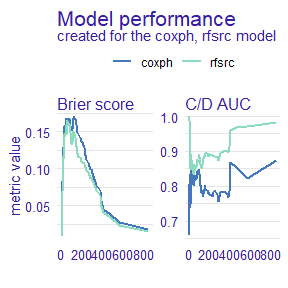We can also plot the scalar metrics in the form of bar plots.

plot(mp_cph, mp_rsf, metrics_type="scalar")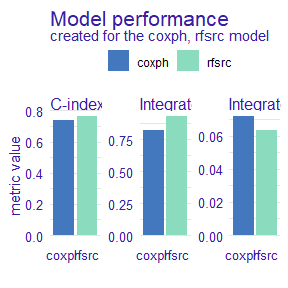From this comparison, we see that the Random Survival Forest model is better in the Brier score metric (lower is better), Cumulative/Dynamic AUC metric (higher is better), and their integrated versions, as well as when we consider the concordance index. Therefore, it is a better candidate for making predictions but lacks interpretability compared to the Proportional Hazards model.

# Global explanations

## Variable importance

Next, we check how each variable influences the models’ predictions on a global level. For this purpose, we use the model_parts() function. It calculates permutational variable importance with the difference being that the loss function is time-dependent (by default it is loss_brier_score()), so the influence of each variable can be different at each considered time point.

model_parts_rsf <- model_parts(rsf_exp)
model_parts_cph <- model_parts(cph_exp)

plot(model_parts_cph,model_parts_rsf)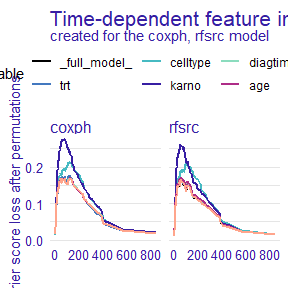For both models, the permutation of the karno variable leads to the highest increase in the loss function, with the second being celltype. These two variables are the most important for models making predictions.

We can use another loss function to ensure this observation is consistent. Let’s use loss_one_minus_cd_auc(), but let’s also change the plot type to show the difference between the loss function after a given variable’s permutation and the loss of the full model with all variables. This means that the values on the y-axis represent only the change in the loss function after permuting each variable.

model_parts_rsf_auc <- model_parts(rsf_exp, loss_function=loss_one_minus_cd_auc, type="difference")
model_parts_cph_auc <- model_parts(cph_exp, loss_function=loss_one_minus_cd_auc, type="difference")

# NOTE: this may take a long time, so a progress bar is available. To enable it, use:
# progressr::with_progress(model_parts(rsf_exp, loss_function=loss_one_minus_cd_auc, type="difference"))

plot(model_parts_cph_auc,model_parts_rsf_auc)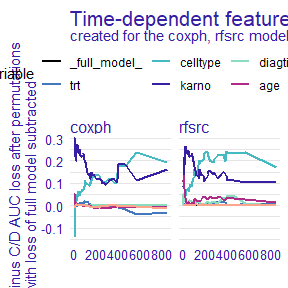We observe that the results are consistent with loss_brier_score(). That’s good news - a simple sanity check that these variables are the most important for these models.

## Partial dependence

The next type of global explanation this package provides is partial dependence plots. This is calculated using the model_profile() function. These plots show how setting one variable to a different value would, on average, influence the model’s prediction. Again, this is an extension of partial dependence known from regression and classification tasks, applied to survival models by extending it to take the time dimension into account.

Note that we need to set the categorical_variables parameter in order to avoid nonsensical values, such as the treatment value of 0.5. All factors are automatically detected as categories, but if you want to treat a numerical variable as a categorical one, you need to set it here.

model_profile_cph <- model_profile(cph_exp, categorical_variables=c("trt", "prior"))
plot(model_profile_cph, facet_ncol = 1)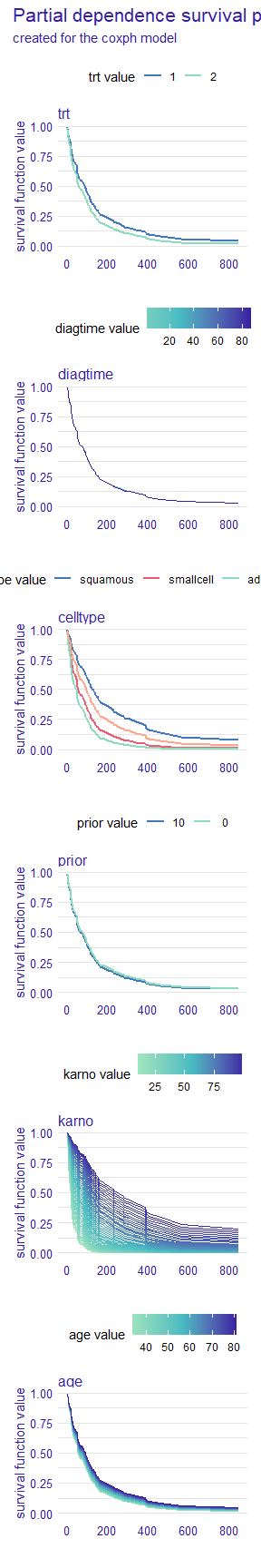From the plot, we see that for the proportional hazards model, the prior and diagtime variables are not very important. The plotted bands are very thin, almost overlapping, so the overall prediction will be similar no matter what value these variables take. On the other hand, the karno variable has a very wide band which means that even a small change in its value causes a big difference in the predicted survival function. We can also see that its lower values indicate a lower chance of survival (survival function decreases quicker).

We can also plot the same information for the random survival forest. This time let’s change the way of plotting numerical variables, instead of the values of the variables being represented by the colors and survival function values on the y-axis, let’s swap them.

model_profile_rsf <- model_profile(rsf_exp, categorical_variables=c("trt", "prior"))
plot(model_profile_rsf, facet_ncol = 1, numerical_plot_type = "contour")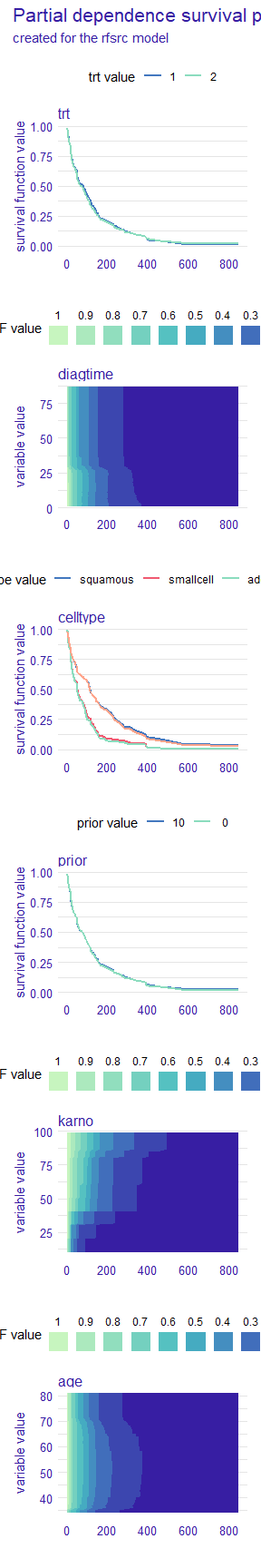This type of plot also gives us valuable insight that is easy to overlook in the other type. For example, we see a sharp drop in survival function values around diagtime=25. We also observe that the most significant influence of the karno variable is consistent across the proportional hazards and random survival forest.

# Local explanations

Another kind of functionality provided by this package is local explanations. The predict_parts() function can be used to assess the importance of variables while making predictions for a selected observation. This can be done by two methods, SurvSHAP(t) and SurvLIME.

### SurvSHAP(t)

SurvSHAP(t) explanations are an extension of SHAP values for survival models. They show a breakdown of the prediction into individual variables.

predict_parts_cph_32 <- predict_parts(cph_exp, veteran[32,])
predict_parts_rsf_32 <- predict_parts(rsf_exp, veteran[32,])
plot(predict_parts_cph_32, predict_parts_rsf_32)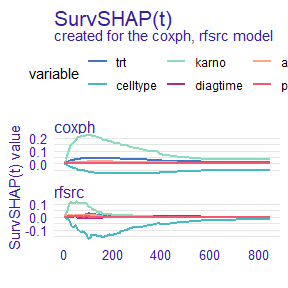predict_parts_cph_12 <- predict_parts(cph_exp, veteran[12,])
predict_parts_rsf_12 <- predict_parts(rsf_exp, veteran[12,])
plot(predict_parts_cph_12, predict_parts_rsf_12)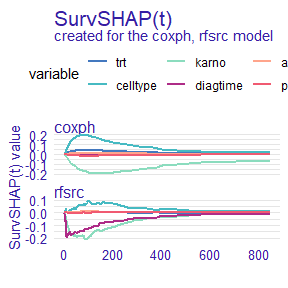On the first plot, we see that for observation 32 from the veteran data set, the value of karno variable improves the chances of survival of this individual. In contrast, the value of the celltype variable decreases them. This is true for both explained models.

On the second plot, it can be seen, that for observation 12, the situation is flipped, celltype increases the chances of survival while karno decreases them. Interestingly, for the random survival forest, one of the most influential variables is diagtime, which the proportional hazards model almost ignores.

### SurvLIME

A different way of attributing variable importance is provided by the SurvLIME method. It works by finding a surrogate proportional hazards model that approximates the survival model at the local area around an observation of interest. Variable importance is then attributed using the coefficients of the found model.

predict_parts_cph_12_lime <- predict_parts(cph_exp, veteran[12,], type="survlime")
predict_parts_rsf_12_lime <- predict_parts(rsf_exp, veteran[12,], type="survlime")
plot(predict_parts_cph_12_lime, type="local_importance")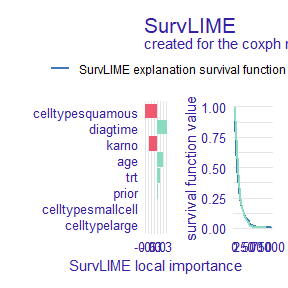plot(predict_parts_rsf_12_lime, type="local_importance")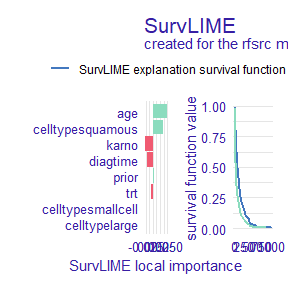The left part of the plot shows which variables are most important and if their value increases or lowers the chances of survival, whereas the right shows the black-box model prediction together with the one from the found surrogate model. This is useful information because the closer these functions are, the more accurate the explanation can be.

## Ceteris paribus

Another explanation technique provided by this package is ceteris paribus profiles. They show how the prediction changes when we change the value of one variable at a time. We can think of them as the equivalent of partial dependence plots but applied to a single observation. The predict_profile() function is used to make these explanations.

predict_profile_cph_32 <- predict_profile(cph_exp, veteran[32,], categorical_variables=c("trt", "prior"))
plot(predict_profile_cph_32, facet_ncol=1)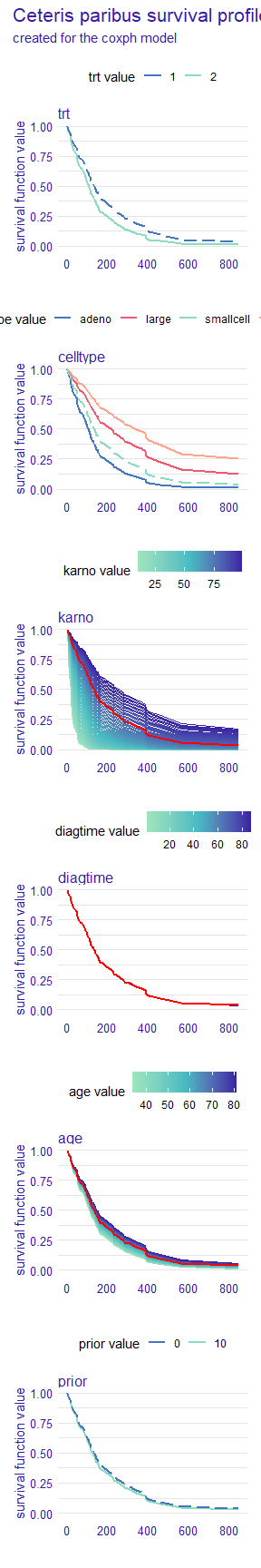predict_profile_rsf_32 <- predict_profile(rsf_exp, veteran[32,], categorical_variables=c("trt", "prior"))
plot(predict_profile_rsf_32, facet_ncol=1)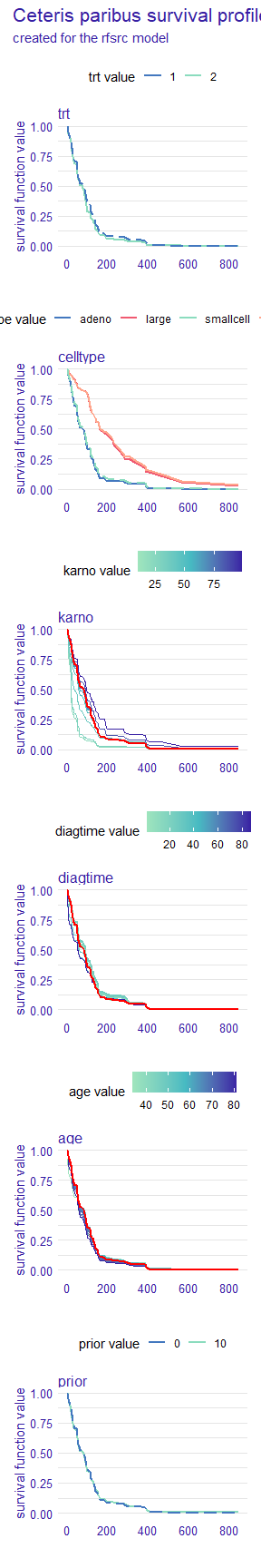These plots also give a lot of valuable insight. For example, we see that the prior variable does not influence the predictions very much, as the lines representing survival functions for its different values almost overlap. We also observe that the celltype values of "small" and "adeno", as well as "large" and "squamous" result in almost the same prediction for this observation. It can be seen that the most important variable for this observation is karno, as the differences in the survival function are the greatest, with low values indicating lower chances of survival. In contrast, high values of the diagtime variable seem to indicate lower chances of survival.